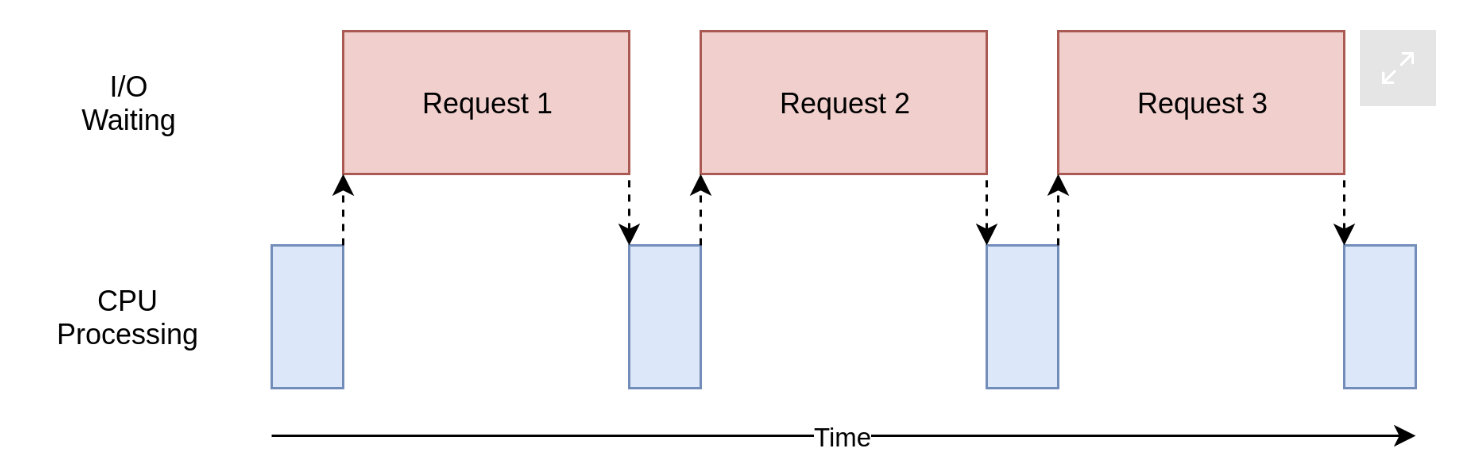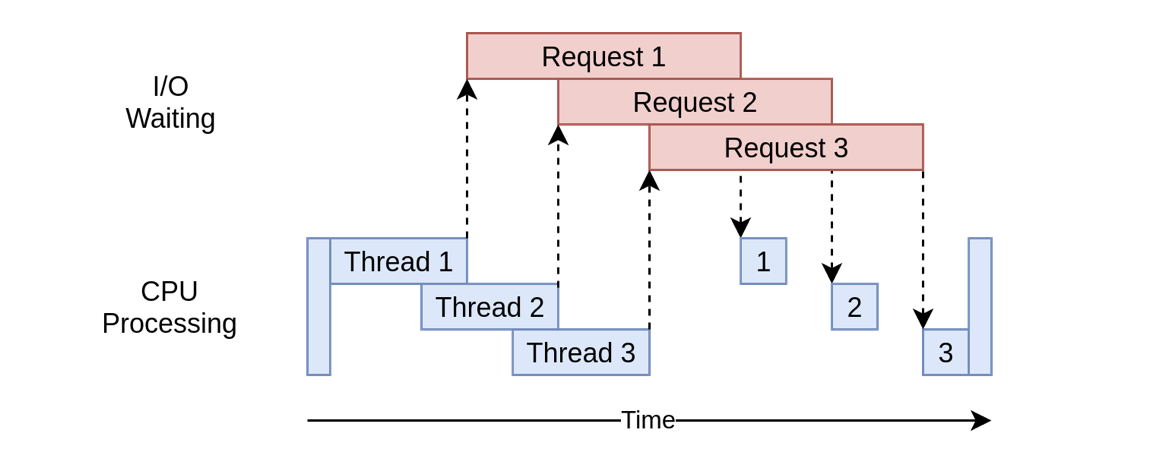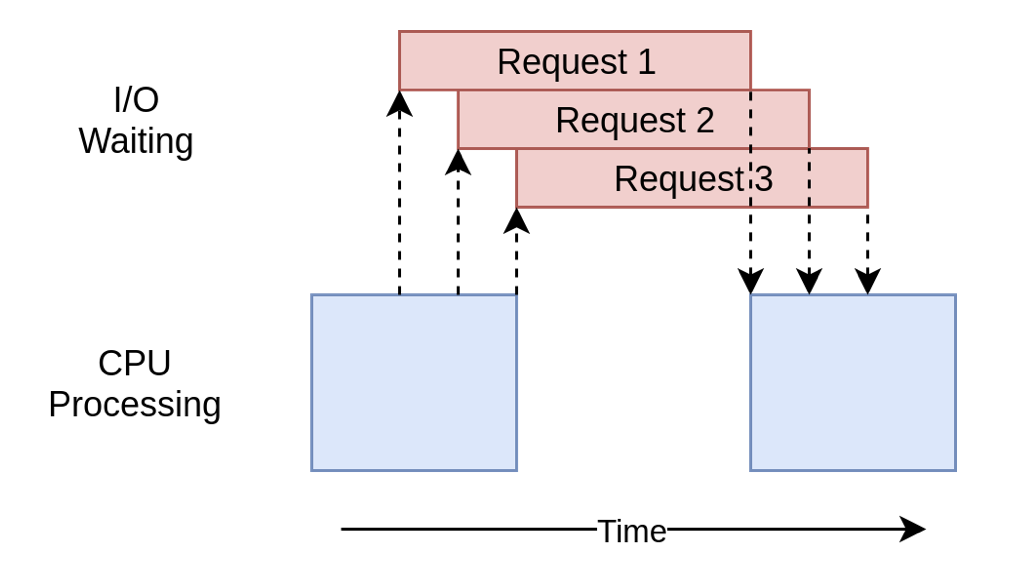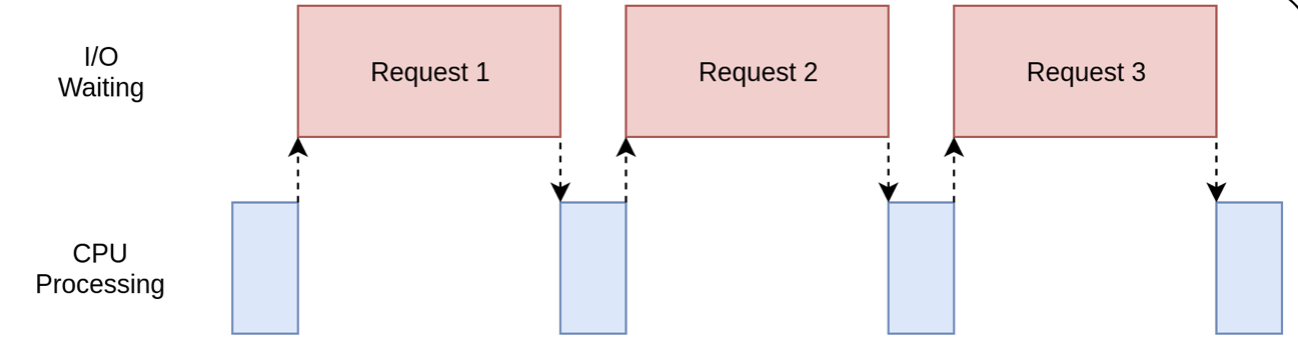## 什么是并发和并行？

Python中并行任务的实现方式是多进程multiprocessing，通过multiprocessing库，Python可以在程序主进程中创建新的子进程。这里的一个进程可以被认为是一个几乎完全不同的程序，尽管从技术上讲，它们通常被定义为资源集合，其中资源包括内存、文件句柄等。换一种说法是，每个子进程都拥有自己的Python解释器，因此，Python中的并行任务可以使用一颗以上的CPU，每一颗CPU都可以跑一个进程，是真正的同时运行，而不需要切换，如此Python就可以完成并行任务。

## 什么时候使用并发？IO密集型任务``````import requests
import time

with session.get(url) as response:
print(f"下载了{len(response.content)}行数据")

with requests.Session() as session:
for url in sites:

if __name__ == "__main__":

sites = ["//v3u.cn"] * 50
start_time = time.time()
duration = time.time() - start_time
print(f"下载了 {len(sites)}次，执行了{duration}秒")
``````

``````下载了76347行数据

[Finished in 9.6s]
``````

``````import concurrent.futures
import requests
import time

def get_session():

session = get_session()
with session.get(url) as response:
print(f"下载了{len(response.content)}行数据")

if __name__ == "__main__":

sites = ["//v3u.cn"] * 50
start_time = time.time()
duration = time.time() - start_time
print(f"下载了 {len(sites)}次，执行了{duration}秒")
``````

``````下载了76424行数据

````````````pip3 install aiohttp
``````

``````import asyncio
import time
import aiohttp

async with session.get(url) as response:
print(f"下载了{response.content_length}行数据")

async with aiohttp.ClientSession() as session:
for url in sites:

if __name__ == "__main__":
sites = ["//v3u.cn"] * 50
start_time = time.time()
duration = time.time() - start_time
print(f"下载了 {len(sites)}次，执行了{duration}秒")
``````

``````

``````## 并行方式：多进程multiprocessing

``````import requests
import multiprocessing
import time

session = None

def set_global_session():
global session
if not session:
session = requests.Session()

with session.get(url) as response:
name = multiprocessing.current_process().name
print(f"读了{len(response.content)}行")

with multiprocessing.Pool(initializer=set_global_session) as pool:

if __name__ == "__main__":
sites = ["//v3u.cn"] * 50
start_time = time.time()
duration = time.time() - start_time
print(f"下载了 {len(sites)}次，执行了{duration}秒")
``````

``````读了76000行

``````## 并行方式的选择：CPU密集型任务

``````import time

def cpu_bound(number):
return sum(i * i for i in range(number))

def find_sums(numbers):
for number in numbers:
cpu_bound(number)

if __name__ == "__main__":
numbers = [5_000_000 + x for x in range(20)]
start_time = time.time()
find_sums(numbers)
duration = time.time() - start_time
print(f"{duration}秒")
``````

``````4.466595888137817秒
``````

``````import multiprocessing
import time

def cpu_bound(number):
return sum(i * i for i in range(number))

def find_sums(numbers):
with multiprocessing.Pool() as pool:
pool.map(cpu_bound, numbers)

if __name__ == "__main__":
numbers = [5_000_000 + x for x in range(20)]

start_time = time.time()
find_sums(numbers)
duration = time.time() - start_time
print(f"{duration}秒")
``````

``````1.1755797863006592秒
``````

``````import concurrent.futures
import time

def cpu_bound(number):
return sum(i * i for i in range(number))

def find_sums(numbers):
executor.map(cpu_bound, numbers)

if __name__ == "__main__":
numbers = [5_000_000 + x for x in range(20)]

start_time = time.time()
find_sums(numbers)
duration = time.time() - start_time
print(f"{duration}秒")
``````

``````4.452666759490967秒
``````